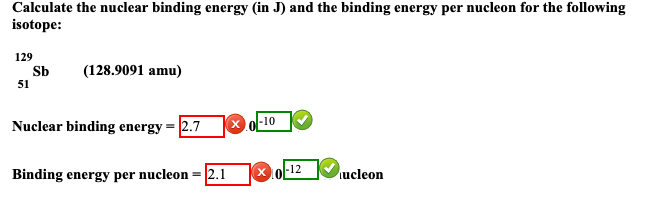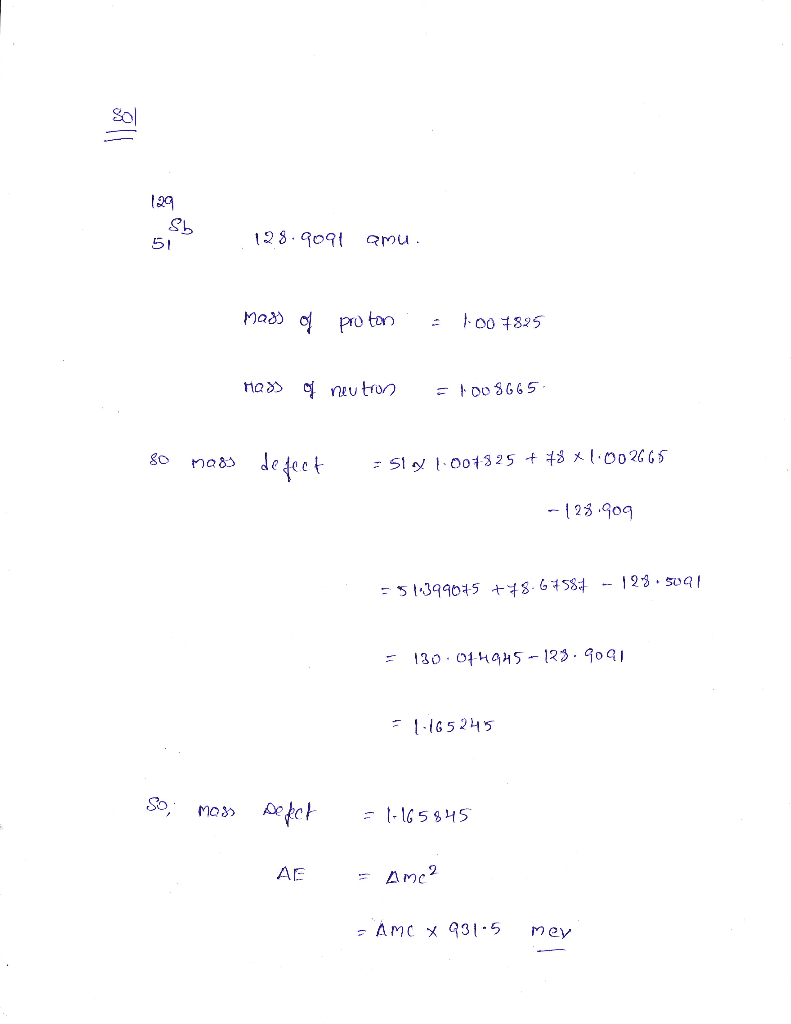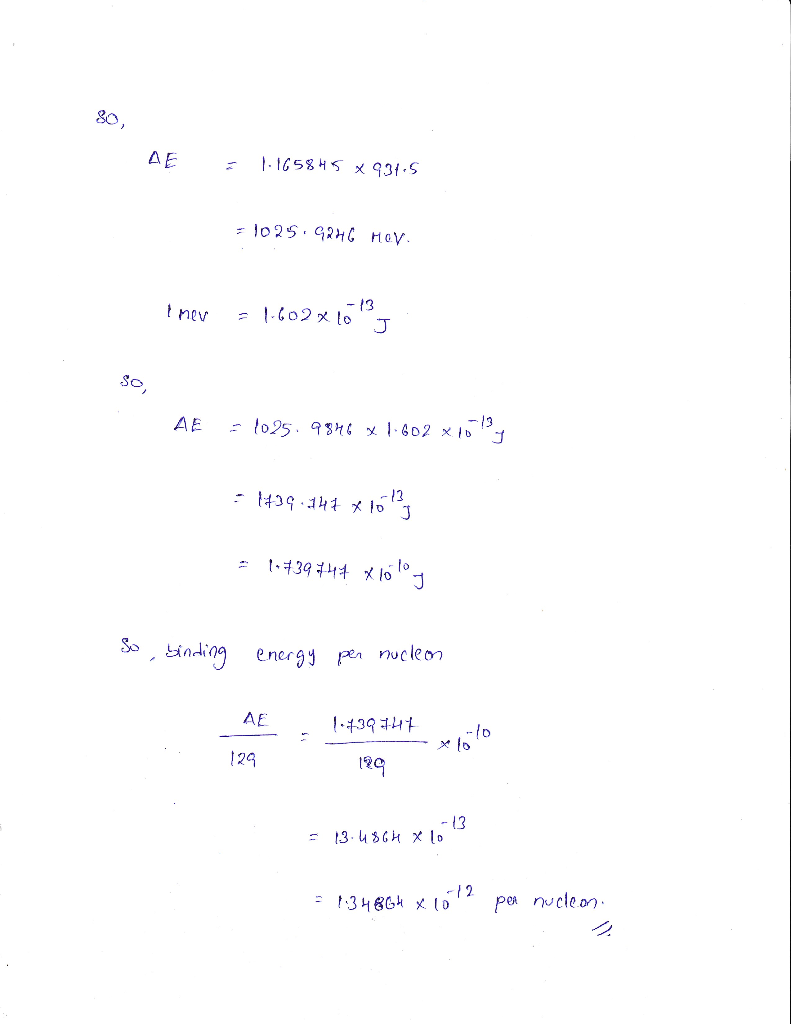# Calculate the nuclear binding energy (in J) and the binding energy per nucleon for the following isotope: 129 Sb (128.9091 amu) 51 10 Nuclear binding energy 27 12 Binding energy per nucleon-2.1 ucleo...Calculate the nuclear binding energy (in J) and the binding energy per nucleon for the following isotope: 129 Sb (128.9091 amu) 51 10 Nuclear binding energy 27 12 Binding energy per nucleon-2.1 ucleon##### Add Answer of: Calculate the nuclear binding energy (in J) and the binding energy per nucleon for the following isotope: 129 Sb (128.9091 amu) 51 10 Nuclear binding energy 27 12 Binding energy per nucleon-2.1 ucleo...
More Homework Help Questions Additional questions in this topic.